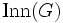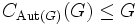# Centerless implies NSCFN in automorphism group

Jump to: navigation, search

## Statement

Suppose$G$ is a Centerless group (?). Then, consider the homomorphism$G \to \operatorname{Aut}(G)$, given by the action by conjugation. Then, the homomorphism is injective. Identifying$G$ with its image,$\operatorname{Inn}(G)$, we obtain that$G$ is a subgroup of$\operatorname{Aut}(G)$. This subgroup is a NSCFN-subgroup (?), i.e.:

1.$G$ is a normal subgroup inside$\operatorname{Aut}(G)$.
2.$G$ is a self-centralizing subgroup inside$\operatorname{Aut}(G)$, i.e.,$C_{\operatorname{Aut}(G)}(G) \le G$ (in fact,$G$ is a centralizer-free subgroup).
3.$G$ is a fully normalized subgroup of$\operatorname{Aut}(G)$: Every automorphism of$G$ extends to an inner automorphism of$\operatorname{Aut}(G)$.